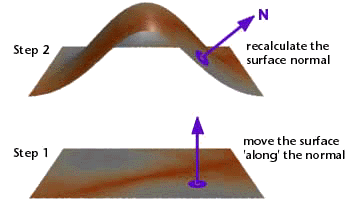### RSLWhat is a Displacement Shader?

Displacement shaders alter the smoothness of a surface, however, unlike bump mapping which mimics the appearance of bumpiness by reorientating surface normals, displacement shading genuinly effects the geometry of a surface. In the case of Pixars prman renderer, each object in a 3D scene is sub-divided into a fine mesh of micro-polygons after which, if a displacement shader has been assigned to an object, they are "pushed" or "pulled" in a direction that is parallel to the original surface normal of the micro-polygon. After displacing the micro-polygon the orientation of the local surface normal(`N`) is recalculated.Figure 1

The following algorithm lists the four basic steps that a displacement shader generally follows in order to set the position (`P`) and normal (`N`) of the micro-polygon being shaded.

 1 Make a copy of the surface normal (`N`) ensuring it is one unit in length. 2 Calculate an appropriate value for the displacement - what will be referred to in these notes as the `hump` factor! 3 Calculate a new position of the surface point "`P`" by moving it "along" the copy of the surface normal by an amount equal to `hump` scaled by the value of the instance variable `Km`. 4 Recalculate the surface normal (`N`).

To make a meaningful decision about the distance, if any, a micro-polygon should be displaced, a shader will make reference to the micro-polygon's,

• 2D surface position `s`, `t`, `u`, `v`,
• 3D xyz position `P`,
• orientation `N`,
• camera distance `L`.

plus other less obvious attributes of a micro-polygon. Such information is either directly or indirectly available in data the renderer makes available to a shader through the use of global variables.

#### Displacement Shading & Global Variables

The following table lists the global variables accessible to a displacement shader. [List of global variables available to surface shaders].

 Global variable P N s, t Ng u,v du, dv dPdu,dPdv I E Meaning surface position surface geometric normal surface texture coordinates surface geometric normal surface parameters change in u, v across the surface change in position with u and v camera viewing direction position of the camera